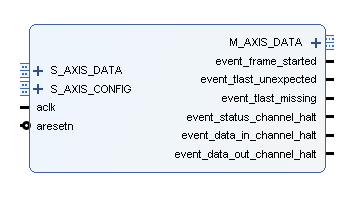# Vivado Xilinx FFT IP核v9.0 使用详解（附仿真实例）

Vivado Xilinx FFT IP核v9.0 使用详解（附仿真实例）

1.输入输出端口如上图所示，左侧的端口均为输入端口，右侧端口均为输出端口，其中，S_AXIS_DATA为输入数据端口，我们要进行FFT的数据需要通过这根线输入给IP核；S_AXIS_CONFIG为输入配置端口，这个信号包含了对数据进行FFT还是IFFT、缩放因子、FFT变换点数等信息；FFT变换后的数据从M_AXIS_DATA端口输出。这些端口的具体功能可以参见pg109手册。

2.Vivado中IP核的配置

S_AXIS_DATA_TDATA：共32位，其中低16位为输入数据的实部，高16位为输入数据的虚部（但在实际使用中，高16位才是实部，低16位是虚部，如果有大神明白是咋回事儿，欢迎留言）S_AXIS_CONFIG_TDATA：最低位第0位，决定对数据进行FFT还是IFFT，置1时FFT，清零时IIFT，由于要进行补零操作，因此在最终写入S_AXIS_CONFIG_TDATA时，除了最低位以外，还要再补七个零，补到8位M_AXIS_DATA_TDATA：48位数据输出，低24位为实部，高24位为虚部
3.软件仿真

IP核配置完成后，下面开始编写我们的TestBench文件。 我们通过matlab对F(t) = 200 + 100cos(2pi10t) + 100cos(2pi30t) 这个信号以Fs = 100HZ进行采样，采样点数N = 128，采样完成后，将数据转换为16位二进制，并存入txt文件中。matlab程序如下：

clear
Fs=100; %采样率1ns一个点
%t=0:1/Fs:63/Fs; %数据时长：64个采样周期
N = 128;
n = 1:N;
t = n/Fs;
% 生成测试信号
f1 = 10; %
f2 = 30; %
s1 = cos(2*pi*f1*t);
s2 = cos(2*pi*f2*t);
signalN = 2 + s1 + s2 ;
data_before_fft = 100*signalN; %系数放大100倍
fp = fopen('D:ynq_Coredata_before_fft.txt','w');
for i = 1:N
if(data_before_fft(i)>=0)
temp= dec2bin(data_before_fft(i),16);
else
temp= dec2bin(data_before_fft(i)+2^16+1, 16);
end
for j=1:16
fprintf(fp,'%s',temp(j));
end
fprintf(fp,' ');
end
fclose(fp);
y = fft(data_before_fft,N);
y = abs(y);
f = n*Fs/N;
plot(f,y);

TB文件代码如下：

`timescale 1ns / 1ps
module FFT_test2();
reg clk;
reg rst_n;
reg signed [15:0] Time_data_I[127:0];
reg data_finish_flag;
wire fft_s_config_tready;
reg signed [31:0] fft_s_data_tdata;
reg fft_s_data_tvalid;
wire fft_s_data_tready;
reg fft_s_data_tlast;
wire signed [47:0] fft_m_data_tdata;
wire signed [7:0] fft_m_data_tuser;
wire fft_m_data_tvalid;
reg fft_m_data_tready;
wire fft_m_data_tlast;
wire fft_event_frame_started;
wire fft_event_tlast_unexpected;
wire fft_event_tlast_missing;
wire fft_event_status_channel_halt;
wire fft_event_data_in_channel_halt;
wire fft_event_data_out_channel_halt;
reg [7:0] count;
reg signed [23:0] fft_i_out;
reg signed [23:0] fft_q_out;
reg signed [47:0] fft_abs;
initial begin
clk = 1'b1;
rst_n = 1'b0;
fft_m_data_tready = 1'b1;
\$readmemb("D:/Zynq_Core/data_before_fft.txt",Time_data_I);
end
always #5 clk = ~clk;
always @ (posedge clk or negedge rst_n) begin
if(!rst_n) begin
fft_s_data_tvalid <= 1'b0;
fft_s_data_tdata <= 32'd0;
fft_s_data_tlast <= 1'b0;
data_finish_flag <= 1'b0;
count <= 8'd0;
rst_n = 1'b1;
end
else if (fft_s_data_tready) begin
if(count == 8'd127) begin
fft_s_data_tvalid <= 1'b1;
fft_s_data_tlast <= 1'b1;
fft_s_data_tdata <= {Time_data_I[count],16'd0};
count <= 8'd0;
data_finish_flag <= 1'b1;
end
else begin
fft_s_data_tvalid <= 1'b1;
fft_s_data_tlast <= 1'b0;
fft_s_data_tdata <= {Time_data_I[count],16'd0};
count <= count + 1'b1;
end
end
else begin
fft_s_data_tvalid <= 1'b0;
fft_s_data_tlast <= 1'b0;
fft_s_data_tdata <= fft_s_data_tdata;
end
end
always @ (posedge clk) begin
if(fft_m_data_tvalid) begin
fft_i_out <= fft_m_data_tdata[23:0];
fft_q_out <= fft_m_data_tdata[47:24];
end
end
always @ (posedge clk) begin
fft_abs <= \$signed(fft_i_out)* \$signed(fft_i_out)+ \$signed(fft_q_out)* \$signed(fft_q_out);
end
//fft ip核例化
xfft_0 u_fft(
.aclk(clk), // 时钟信号（input）
.aresetn(rst_n), // 复位信号，低有效（input）
.s_axis_config_tdata(8'd1), // ip核设置参数内容，为1时做FFT运算，为0时做IFFT运算（input）
.s_axis_config_tvalid(1'b1), // ip核配置输入有效，可直接设置为1（input）
.s_axis_config_tready(fft_s_config_tready), // output wire s_axis_config_tready
//作为接收时域数据时是从设备
.s_axis_data_tdata(fft_s_data_tdata), // 把时域信号往FFT IP核传输的数据通道,[31:16]为虚部，[15:0]为实部（input，主->从）
.s_axis_data_tvalid(fft_s_data_tvalid), // 表示主设备正在驱动一个有效的传输（input，主->从）
.s_axis_data_tready(fft_s_data_tready), // 表示从设备已经准备好接收一次数据传输（output，从->主），当tvalid和tready同时为高时，启动数据传输
.s_axis_data_tlast(fft_s_data_tlast), // 主设备向从设备发送传输结束信号（input，主->从，拉高为结束）
//作为发送频谱数据时是主设备
.m_axis_data_tdata(fft_m_data_tdata), // FFT输出的频谱数据，[47:24]对应的是虚部数据，[23:0]对应的是实部数据(output，主->从)。
.m_axis_data_tuser(fft_m_data_tuser), // 输出频谱的索引(output，主->从)，该值*fs/N即为对应频点；
.m_axis_data_tvalid(fft_m_data_tvalid), // 表示主设备正在驱动一个有效的传输（output，主->从）
.m_axis_data_tready(fft_m_data_tready), // 表示从设备已经准备好接收一次数据传输（input，从->主），当tvalid和tready同时为高时，启动数据传输
.m_axis_data_tlast(fft_m_data_tlast), // 主设备向从设备发送传输结束信号（output，主->从，拉高为结束）
//其他输出数据
.event_frame_started(fft_event_frame_started), // output wire event_frame_started
.event_tlast_unexpected(fft_event_tlast_unexpected), // output wire event_tlast_unexpected
.event_tlast_missing(fft_event_tlast_missing), // output wire event_tlast_missing
.event_status_channel_halt(fft_event_status_channel_halt), // output wire event_status_channel_halt
.event_data_in_channel_halt(fft_event_data_in_channel_halt), // output wire event_data_in_channel_halt
.event_data_out_channel_halt(fft_event_data_out_channel_halt) // output wire event_data_out_channel_halt
);
endmodule

4.仿真分析

## ORM思想

ORM思想publicList<Book>findAll(){Connectionconnection=null;PreparedStatementpreparedStatemen...

## 2. 声明一个实现任意类型数据存取的类模板Store，通过具体数据类型参数对类模板进行实例化，生成类，再由类建立对象S1，S2，S3和d。

#include<iostream>usingnamespacestd;template<classT>//建立一个类模板classStore{public:Store(){}...

## 2020-12-14

JS冒泡数组极简教程（带注释）<!DOCTYPEhtml><html><head><metacharset="UT...